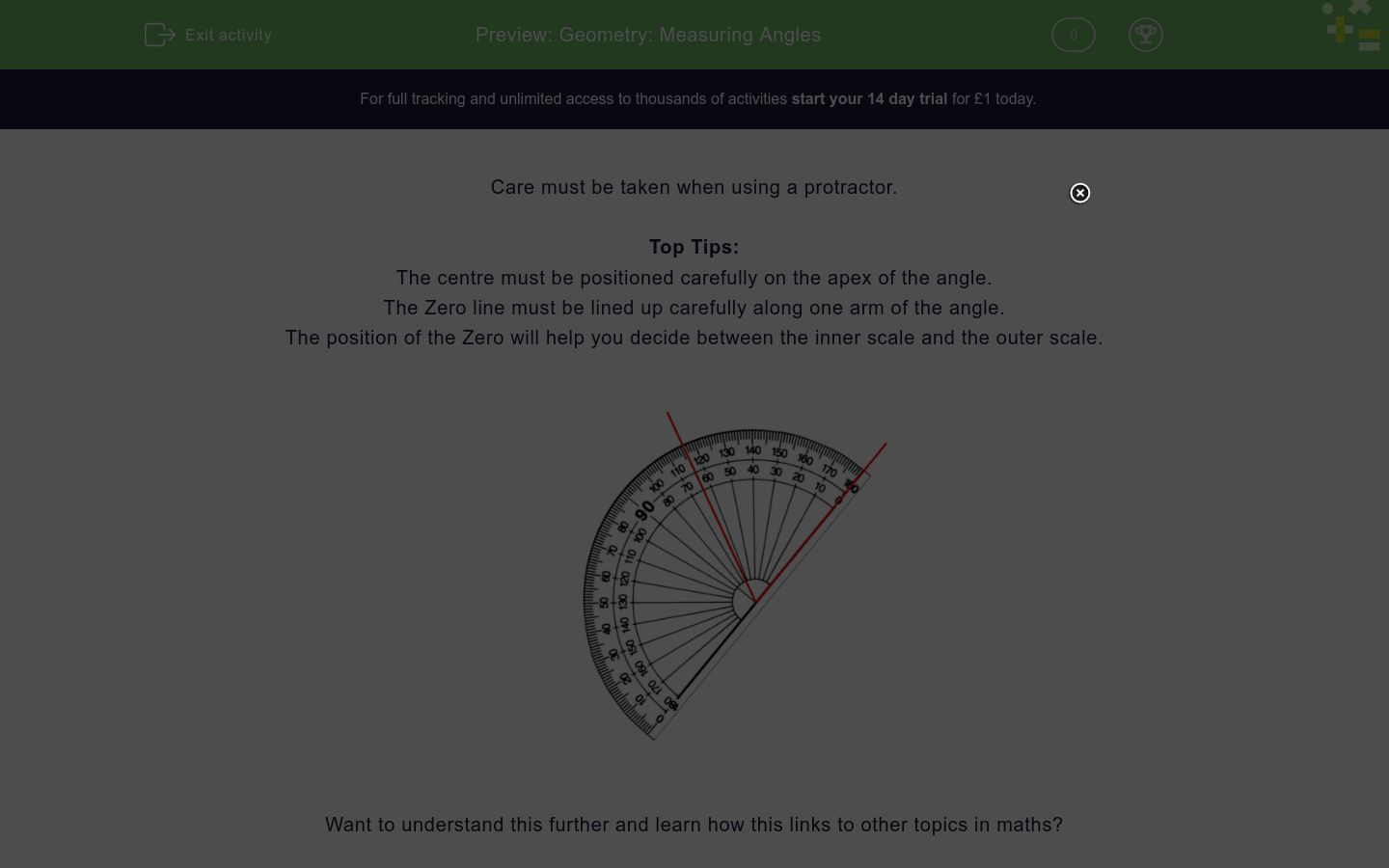# Geometry: Measuring Angles

In this worksheet, students measure angles using the onscreen protractor.Key stage:  KS 2

Curriculum topic:   Geometry: Properties of Shapes

Curriculum subtopic:   Know Angles and Degrees

Difficulty level:### QUESTION 1 of 10

Care must be taken when using a protractor.

Top Tips:

The centre must be positioned carefully on the apex of the angle.

The Zero line must be lined up carefully along one arm of the angle.

The position of the Zero will help you decide between the inner scale and the outer scale.Want to understand this further and learn how this links to other topics in maths?

Why not watch this video?

Use the protractor to measure this angle.

Select the correct angle.70°

110°

180°

Use the protractor to measure this angle.

Select the correct angle.55°

65°

125°

135°

Use the protractor to measure this angle.

Select the correct angle.38°

42°

142°

Use the protractor to measure this angle.

Select the correct angle.80°

100°

180°

Use the protractor to measure this angle.

Select the correct angle.80°

100°

180°

Use the protractor to measure this angle.

Select the correct angle.64°

124°

76°

116°

Use the protractor to measure this angle.

Select the correct angle.65°

75°

115°

125°

Use the protractor to measure this angle.

Select the correct angle.136°

56°

144°

44°

Use the protractor to measure this angle.

Select the correct angle.73°

107°

113°

87°

Use the protractor to measure this angle.

Select the correct angle.48°

152°

32°

148°

• Question 1

Use the protractor to measure this angle.

Select the correct angle.70°
EDDIE SAYS
The angle is acute, so it's less than 90 degrees. The angle is 70 degrees.
• Question 2

Use the protractor to measure this angle.

Select the correct angle.55°
EDDIE SAYS
The angle is acute, so it's less than 90 degrees. The angle is 55 degrees.
• Question 3

Use the protractor to measure this angle.

Select the correct angle.38°
EDDIE SAYS
The angle is acute, so it's less than 90 degrees. The angle is 38 degrees.
• Question 4

Use the protractor to measure this angle.

Select the correct angle.100°
EDDIE SAYS
The angle is obtuse, so it's more than 90 degrees. The angle is 100 degrees.
• Question 5

Use the protractor to measure this angle.

Select the correct angle.80°
EDDIE SAYS
The angle is acute, so it's less than 90 degrees. The angle is 80 degrees.
• Question 6

Use the protractor to measure this angle.

Select the correct angle.116°
EDDIE SAYS
The angle is obtuse, so it's more than 90 degrees. The angle is 116 degrees.
• Question 7

Use the protractor to measure this angle.

Select the correct angle.65°
EDDIE SAYS
The angle is acute, so it's less than 90 degrees. The angle is 65 degrees.
• Question 8

Use the protractor to measure this angle.

Select the correct angle.44°
EDDIE SAYS
The angle is acute, so it's less than 90 degrees. The angle is 44 degrees.
• Question 9

Use the protractor to measure this angle.

Select the correct angle.107°
EDDIE SAYS
The angle is obtuse, so it's more than 90 degrees. The angle is 107 degrees.
• Question 10

Use the protractor to measure this angle.

Select the correct angle.148°
EDDIE SAYS
The angle is obtuse, so it's more than 90 degrees. The angle is 148 degrees.
---- OR ----